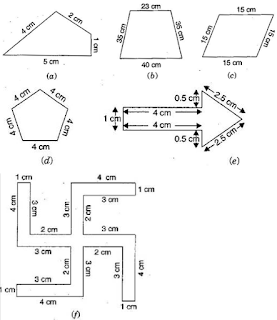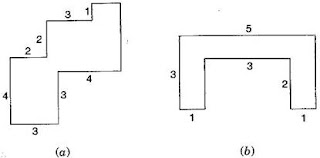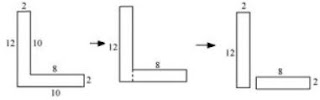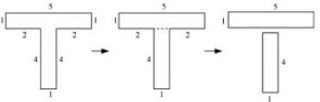# NCERT Solutions for Class 6 Maths Chapter 10 Mensuration

On this page, you will get Chapter 10 Mensuration NCERT Solutions for Class 6 Maths which will help students in performing exceptionally well in their exams. You can download PDF of Class 6 Maths NCERT Solutions Chapter 10 Mensuration through which you can study in an organized manner and outperform your classmates. It help in building a great foundation of concepts and make easy for the students to understand basics.

Chapter 10 NCERT Solutions will help you in understanding the concepts behind the questions. These NCERT Solutions are prepared as per the accordance of latest CBSE guidelines so you can score maximum marks.Exercise 10.1

1. Find the perimeter of each of the following figures:(a) Perimeter = Sum of all the sides
= 4 cm + 2 cm + 1 cm + 5 cm
= 12 cm

(b) Perimeter = Sum of all the sides
= 23 cm + 35 cm + 40 cm + 35 cm
= 133 cm

(c) Perimeter = Sum of all the sides
= 15 cm + 15 cm + 15 cm + 15 cm
= 60 cm

(d) Perimeter = Sum of all the sides
= 4 cm + 4 cm + 4 cm + 4 cm + 4 cm
= 20 cm

(e) Perimeter = Sum of all the sides
= 1 cm + 4 cm + 0.5 cm + 2.5 cm + 2.5 cm + 0.5 cm + 4 cm
= 15 cm

(f) Perimeter = Sum of all the sides
= 4 cm + 1 cm + 3 cm + 2 cm + 3 cm + 4 cm + 1 cm + 3 cm + 2 cm + 3 cm + 4 cm +

1 cm + 3 cm + 2 cm + 3 cm + 4 cm + 1 cm + 3 cm + 2 cm + 3 cm
= 52 cm

2. The lid of a rectangular box of sides 40 cm by 10 cm is sealed all round with tape. What is the length of the tape required?

Total length of tape required = Perimeter of rectangle= 2 ( length + breadth)
= 2 ( 40 + 10)
= 2×50
= 100 cm = 1 m
Thus, the total length of tape required is 100 cm or 1 m.

3. A table-top measures 2 m 25 cm by 1 m 50 cm. What is the perimeter of the table-top?

Length of table top = 2 m 25 cm = 2.25 m
Breadth of table top = 1 m 50 cm = 1.50 m
Perimeter of table top = 2 x (length + breadth)
= 2 × (2.25 + 1.50)
= 2 × 3.75 = 7.50 m
Thus, perimeter of table top is 7.5 m.

4. What is the length of the wooden strip required to frame a photograph of length 32 cm and breadth 21 cm respectively?

Length of wooden strip = Perimeter of photograph
Perimeter of photograph = 2 × (length + breadth)
= 2 (32 + 21)
= 2 × 53 cm = 106 cm
Thus, the length of the wooden strip required is 106 cm.

5. A rectangular piece of land measures 0.7 km by 0.5 km. Each side is to be fenced with 4 rows of wires. What is the length of the wire needed?

Since the 4 rows of wires are needed. Therefore the total length of wires is equal to 4 times the perimeter of rectangle.
Perimeter of rectangular piece of land = 2 × (length + breadth)
= 2 × (0.7 + 0.5)
= 2 × 1.2
= 2.4 km
= 2.4 × 1000 m
= 2400 m
Thus, the length of wire = 4 × 2400 = 9600 m = 9.6 km

6. Find the perimeter of each of the following shapes:
(a) A triangle of sides 3 cm, 4 cm and 5 cm.
(b) An equilateral triangle of side 9 cm.
(c) An isosceles triangle with equal sides 8 cm each and third side 6 cm.

(a) Perimeter of ΔABCΔABC = AB + BC + CA= 3 cm + 5 cm + 4 cm = 12 cm

(b) Perimeter of equilateral ΔABCΔABC = 3×side= 3×9 cm = 27 cm

(c) Perimeter of ΔABCΔABC = AB + BC + CA= 8 cm + 6 cm + 8 cm = 22 cm

7. Find the perimeter of a triangle with sides measuring 10 cm, 14 cm and 15 cm.

Perimeter of triangle = Sum of all three sides
= 10 cm + 14 cm + 15 cm = 39 cm
Thus, perimeter of triangle is 39 cm.

8. Find the perimeter of a regular hexagon with each side measuring 8 cm.

Perimeter of Hexagon = 6 × length of one side
= 6 x 8 m = 48 m
Thus, the perimeter of hexagon is 48 m.

9. Find the side of the square whose perimeter is 20 m.

Perimeter of square = 4 × side
⇒ 20 = 4 × side
⇒ side = 20/4 = 5 cm
Thus, the side of square is 5 cm.

10. The perimeter of a regular pentagon is 100 cm. How long is its each side?

Perimeter of regular pentagon = 100 cm
⇒ 5 × side = 100 cm
⇒ side = 100/5 = 20 cm
Thus, the side of regular pentagon is 20 cm.

11. A piece of string is 30 cm long. What will be the length of each side if the string is used to form:
(a) a square
(b) an equilateral triangle
(c) a regular hexagon?

Length of string = Perimeter of each figure
(a) Perimeter of square = 30 cm
⇒ 4 × side = 30 cm
⇒ side = 30/4 = 7.5 cm
Thus, the length of each side of square is 7.5 cm.

(b) Perimeter of equilateral triangle = 30 cm
⇒ 3 × side = 30 cm
⇒ side = 30/3 = 10 cm
Thus, the length of each side of equilateral triangle is 10 cm.

(c) Perimeter of hexagon = 30 cm
⇒ 6 × side = 30 cm
⇒ side = 30/6 = 5 cm
Thus, the length of each side of hexagon is 5 cm.

12. Two sides of a triangle are 12 cm and 14 cm. The perimeter of the triangle is 36 cm. What is the third side?

Let the length of third side be xx cm.
Length of other two side are 12 cm and 14 cm.
Now, Perimeter of triangle = 36 cm
⇒ 12+14+x = 36
⇒ 26+x = 6
⇒ x = 36−26
⇒ x=10
Thus, the length of third side is 10 cm.

13. Find the cost of fencing a square park of side 250 m at the rate of Rs 20 per meter.

Side of square = 250 m
Perimeter of square = 4 × side
= 4 × 250 = 1000 m
Since, cost of fencing per meter = Rs. 20
Therefore, cost of fencing of 1000 meters = 20×1000 = Rs. 20,000

14. Find the cost of fencing a rectangular park of length 175 m and breadth 125 m at the rate of Rs. 12 per meter.

Length of rectangular park = 175 m
Breadth of rectangular park = 125 m
Perimeter of park = 2 x (length + breadth)
= 2 × (175 + 125)
= 2×300 = 600 m
Since, cost of fencing park per meter = Rs. 12
Therefore, cost of fencing park of 600 m = 12×600 = Rs. 7,200

15. Sweety runs around a square park of side 75 m. Bulbul runs around a rectangular park with length of 60 m and breadth 45 m. Who covers less distance?

Distance covered by Sweety = Perimeter of square park
Perimeter of square = 4×side
= 4×75 = 300 m
Thus, distance covered by Sweety is 300 m.
Now, distance covered by Bulbul = Perimeter of rectangular park
Perimeter of rectangular park = 2×(length + breadth)
= 2×(60 + 45)
= 2×105 = 210 m
Thus, Bulbul covers the distance of 210 m.
So, Bulbul covers less distance.

16. What is the perimeter of each of the following figures? What do you infer from the answer?(a) Perimeter of square = 4×side
= 4×25 = 100 cm

(b) Perimeter of rectangle = 2×(length + breadth)
= 2×(40 + 10)
= 2×50 = 100 cm

(c) Perimeter of rectangle = 2×(length + breadth)
= 2×(30 + 20)
= 2×50 = 100 cm

(d) Perimeter of triangle = Sum of all sides
= 30 cm + 30 cm + 40 cm = 100 cm
Thus, all the figures have same perimeter.

17. Avneet buys 9 square paving slabs, each with a side 1212 m. He lays them in the form of a square(a) What is the perimeter of his arrangement?
(b) Shari does not like his arrangement. She gets him to lay them out like a cross. What is the
perimeter of her arrangement?
(c) Which has greater perimeter?
(d) Avneet wonders, if there is a way of getting an even greater perimeter. Can you find a way of doing this? (The paving slabs must meet along complete edges, i.e., they cannot be broken.)

(a) 6 m
(b) 10 m
(c) Second arrangement has greater perimeter.
(d) Yes, if all the squares are arranged in row, the perimeter be 10 cm.

Exercise 10.2

1. Find the areas of the following figures by counting squares:(a) Number of filled square = 9
Area covered by squares = 9 × 1 = 9 sq. units

(b) Number of filled squares = 5
Area covered by filled squares = 5 × 1 = 5 sq. units

(c) Number of full filled squares = 2
Number of half filled squares = 4
Area covered by full filled squares = 2 × 1 = 2 sq. units
And, Area covered by half filled squares = 1/2 ×4 = 2 sq. units
Total area = 2 + 2 = 4 sq. units

(d) Number of filled squares = 8
Area covered by filled squares = 8 × 1 = 8 sq. units

(e) Number of filled squares = 10
Area covered by filled squares = 10 × 1 = 10 sq. units

(f) Number of full filled squares = 2
Number of half filled squares = 4
Area covered by full filled squares = 2 × 1 = 2 sq. units
And Area covered by half filled squares = 1/2 ×4 = 2 sq. units
Total area = 2 + 2 = 4 sq. units

(g) Number of full filled squares = 4
Number of half filled squares = 4
Area covered by full filled squares = 4 × 1 = 4 sq. units
And, Area covered by half filled squares = 1/2 ×4 = 2 sq. units
Total area = 4 + 2 = 6 sq. units

(h) Number of filled squares = 5
Area covered by filled squares = 5 × 1 = 5 sq. units

(i) Number of filled squares = 9
Area covered by filled squares = 9 × 1 = 9 sq. units

(j) Number of full filled squares = 2
Number of half filled squares = 4
Area covered by full filled squares = 2 × 1 = 2 sq. units
And Area covered by half filled squares = 1/2 ×4 = 2 sq. units
Total area = 2 + 2 = 4 sq. units

(k) Number of full filled squares = 4
Number of half filled squares = 2
Area covered by full filled squares = 4 × 1 = 4 sq. units
And Area covered by half filled squares = 1/2 ×2 = 1 sq. units
Total area = 4 + 1 = 5 sq. units

(l) Number of full filled squares = 3
Number of half filled squares = 10
Area covered by full filled squares = 3 × 1 = 3 sq. units
And Area covered by half filled squares = 1/2 ×10 = 5 sq. units
Total area = 3 + 5 = 8 sq. units

(m) Number of full filled squares = 7
Number of half filled squares = 14
Area covered by full filled squares = 7 × 1 = 7 sq. units
And Area covered by half filled squares = 1/2 ×14 = 7 sq. units
Total area = 7 + 7 = 14 sq. units

(n) Number of full filled squares = 10
Number of half filled squares = 16
Area covered by full filled squares = 10 × 1 = 10 sq. units
And Area covered by half filled squares = 1/2 ×16 = 8 sq. units
Total area = 10 + 8 = 18 sq. units

Exercise 10.3

1. Find the areas of the rectangles whose sides are:
(a) 3 cm and 4 cm
(b) 12 m and 21 m
(c) 2 km and 3 km
(d) 2 m and 70 cm

(a) Area of rectangle = length × breadth
= 3 cm × 4 cm = 12 cm2

(b) Area of rectangle = length × breadth
= 12 m × 21 m = 252 m2

(c) Area of rectangle = length × breadth
= 2 km × 3 km = 6 km2

(d) Area of rectangle = length × breadth
= 2 m × 70 cm = 2 m × 0.7 m = 1.4 m2

2. Find the areas of the squares whose sides are:
(a) 10 cm (b) 14 cm (c) 5 m

(a) Area of square = side × side
= 10 cm × 10 cm = 100 cm2

(b) Area of square = side x side
= 14 cm × 14 cm = 196 cm2

(c) Area of square = side x side
= 5 m × 5 m = 25 m2

3. The length and the breadth of three rectangles are as given below:
(a) 9 m and 6 m
(b) 17 m and 3 m
(c) 4 m and 14 m
Which one has the largest area and which one has the smallest?

(a) Area of rectangle = length × breadth
= 9 m × 6 m = 54 m2

(b) Area of rectangle = length × breadth
= 3 m × 17 m = 51 m2

(c) Area of rectangle = length x breadth
= 4 m × 14 m = 56 m2
Thus, the rectangle (c) has largest area, i.e. 56 m2 and rectangle (b) has smallest area, i.e., 51 m2.

4. The area of a rectangular garden 50 m long is 300 m2, find the width of the garden.

Length of rectangle = 50 m and Area of rectangle = 300 m2
Since, Area of rectangle = length x breadth
Therefore, Breadth ==300/50 = 6 m
Thus, the breadth of the garden is 6 m.

5. What is the cost of tiling a rectangular plot of land 500 m long and 200 m wide at the rate of Rs. 8 per hundred sq. m?

Length of land = 500 m and Breadth of land = 200 m
Area of land = length x breadth = 500 m × 200 m = 1,00,000 m2
∵ Cost of tiling 100 sq. m of land = Rs. 8
∴ Cost of tilling 1,00,000 sq. m of land = 8/100 ×100000 = Rs. 8000

6. A table-top measures 2 m by 1 m 50 cm. What is its area in square meters?

Length of table = 2 m and breadth of table = 1 m 50 cm = 1.50 m
Area of table = length × breadth
= 2 m × 1.50 m = 3 m2

7. A room is 4 m long and 3 m 50 cm wide. How many square meters of carpet is needed to cover the floor of the room?

Length of room = 4 m and breadth of room = 3 m 50 cm = 3.50 m
Area of carpet = length × breadth
= 4 × 3.50 = 14m2
Therefore, 14m2 of carpet required to cover the floor.

8. A floor is 5 m long and 4 m wide. A square carpet of sides 3 m is laid on the floor. Find the area of the floor that is not carpeted.

Length of floor = 5 m and breadth of floor = 4 m
Area of floor = length × breadth
= 5 m × 4 m = 20 m2
Now, Side of square carpet = 3 m
Area of square carpet = side x side = 3 × 3 = 9 m2
Area of floor that is not carpeted = 20 m2 – 9 m2 = 11 m2

9. Five square flower beds each of sides 1 m are dug on a piece of land 5 m long and 4 m wide. What is the area of the remaining part of the land?

Side of square bed = 1 m
Area of square bed = side × side = 1 m × 1 m = 1 m2
∴ Area of 5 square beds = 1 × 5 = 5 m2
Now, Length of land = 5 m and breadth of land = 4 m
∴ Area of land = length × breadth = 5 m × 4 m = 20 m2
Area of remaining part = Area of land – Area of 5 flower beds
= 20 m2 – 5 m2 = 15 m2

10. By splitting the following figures into rectangles, find their areas. (The measures are given in centimeters)(a) The given figure can be broken into rectangles asArea of 1st rectangle = 12 × 2 = 24 cm2
Area of 2nd rectangle = 8 × 2 = 16 cm2
Total area of the figure = 24 + 16 = 40 cm2

(b) The given figure can be broken into rectangles as followArea of 1st rectangle = 21 × 7  = 147 cm2
Area of 1st square = 7 × 7 = 49 cm2
Area of 2nd square = 7 × 7 = 49 cm2
Total area of the figure = 147 + 49 + 49 = 245 cm2

(c) The given figure can be broken into rectangles as followArea of 1st rectangle = 5 × 1 = 5 cm2
Area of 2nd rectangle = 4 × 1 = 4 cm2
Total area of the figure = 5 + 4 = 9 cm2

12. How many tiles whose length and breadth are 12 cm and 5 cm respectively will be needed to fit in a rectangular region whose length and breadth are respectively:
(a)100 cm and 144 cm
(b)70 cm and 36 cm## NCERT Solutions for Class 6 Maths Chapter 10 Mensuration

Mensuration is a branch of mathematics which study various geometrical shapes, their length, breadth, volume, and area for 2D as well as 3D shapes. NCERT Solutions will help you get a deeper understanding of various topics.

• A figure with no open ends is a closed figure. A closed figure in which all the sides and angles equal are called regular closed figures.

• Exercise 10.1 Chapter 10 Class 6 Maths NCERT Solutions
• Exercise 10.2 Chapter 10 Class 6 Maths NCERT Solutions
• Exercise 10.3 Chapter 10 Class 6 Maths NCERT Solutions

Syllabus is not only difficult but quite lengthy too thus NCERT Solutions will help you in covering those essential topics. It can serve as beneficial tool that can be used to recall various questions any time.

### NCERT Solutions for Class 6 Maths Chapters:

 Chapter 1 Knowing Our Numbers Chapter 2 Whole Numbers Chapter 3 Playing with Numbers Chapter 4 Basic Geometrical Ideas Chapter 5 Understanding Elementary Shapes Chapter 6 Integers Chapter 7 Fractions Chapter 8 Decimals Chapter 9 Data Handling Chapter 11 Algebra Chapter 12 Ratio and Proportion Chapter 13 Symmetry Chapter 14 Practical Geometry

FAQ on Chapter 10 Mensuration

#### How many exercises in Chapter 10 Mensuration?

Chapter 10 Mensuration has three exercises which will allow the students to evaluate their learning almost immediately. Your marks play an important role in shaping future thus these NCERT Solutions will become your comprehensive guide in easy learning and evaluating yourself.

#### What is Perimeter of a rectangle?

Perimeter of a rectangle = Length(l) + Breadth(b) +Length(l) + Breadth (b) = 2 (l + b).

#### What is an equilateral triangle and its perimeter?

A triangle with all its sides and angles equal is called an equilateral triangle. The perimeter of an equilateral triangle with the side 'a' =a+a+a =3 × a.

#### What is area of a rectangle?

Area of a rectangle = length × breadth.